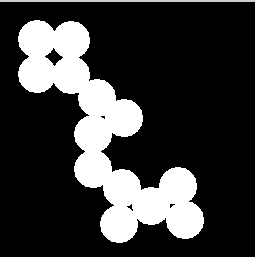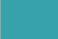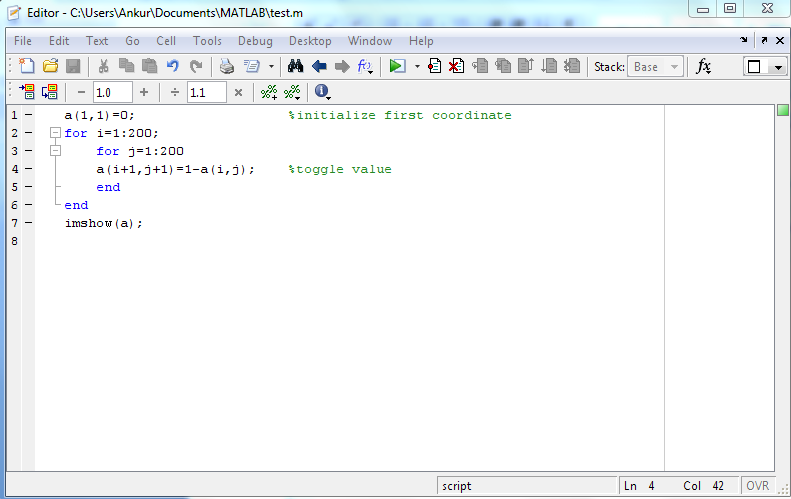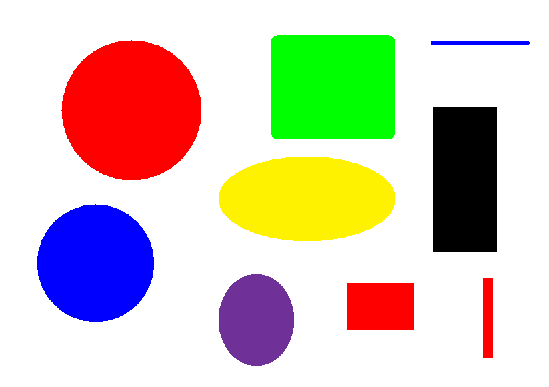# Taking up Images

Posted by SUYOG PATIL on 6:41 PM in , , ,
Taking up Images
Important terms and types of Images
Pixel: Pixels are the building blocks of an image. In other words, a pixel is the smallest possible image that can be depicted on your screen.
Binary Image: An image that consists of only black and white pixels.j
A Binary ImageA Grayscale Image

Grayscale Image: It contains intensity values ranging from a minimum (depicting absolute black) to a maximum (depicting absolute white) and in between varying shades of gray. Typically, this range is between 0 and 255.
*Note:A Color Image: In daily language what we refer to as black-and-white (as in old photos) are actually grayscale. Hence avoid confusion here in technical terms.
Color Image: We all have seen this! Such an image is composed of the three primary colors, Red, Green and Blue, hence also called an RGB image.A Color Image

RGB value: All colors which we see around us can be made by adding red, blue and green components in varying proportions. Hence, any color of the world can uniquely be described by its RGB value, which stands for Red, Blue and Green values. This triplet has each value ranging from 0 to 255, with 0 obviously meaning no component of that particular color and 255 meaning full component. For example, pure red color has RGB value [255 0 0], pure white has [255 255 255], pure black has [0 0 0] andhas RGB value [55 162 170].

Representation of an Image in MATLAB
An image in MATLAB is stored as a 2D matrix (of size mxn) where each element of the matrix represents the intensity of light/color of that particular pixel. Hence, for a binary image, the value of each element of the matrix is either 0 or 1 and for a grayscale image each value lies between 0 and 255. A color image is stored as an mxnx3 matrix where each element is the RGB value of that particular pixel (hence it’s a 3D matrix). You can consider it as three 2D matrices for red, green and blue intensities.

Syntax: IM=imread(‘filename’) where IM is a matrix.
If the file is in the current directory (as described above), then you only need to write the filename, else you need to write the complete path. Filename should be with extension (.jpg, .bmp,..). There are some default images of MATLAB like ‘peppers.png’, ‘cameraman.tif’, etc. You can try reading them as >>im=imread('peppers.png');
It is always advised to use a semi-colon (;) at the end of the statement of reading an image, otherwise… you can try yourself what happens! imshow(): Displays the image.
Syntax: imshow(‘filename’) or imshow(im)
Example, >>imshow('cameraman.tif');

OK, now let’s make our own image, try this:
>>a(1,1)=0;
>>for i=1:200;
for j=1:200
a(i+1,j+1)=1-a(i,j);
end
end
>>imshow(a);

You try out making many different types of images like this just to make yourself comfortable with the commands learnt till now. Data cursor: To see the values of the colors in the figure window, go to Tools>Data Cursor (or select from the toolbar), and click over any point in the image. You can see the RGB values of the pixel at location (X,Y).A better option of data cursor is the function imtool(). Type the following >>imtool(‘peppers.png’); and see the pixel info on lower left corner as you move mouse pointer over different pixels.

Making M-files and functions
M-file
It is a provision in MATLAB where you can execute multiple commands using a single statement. Here the group of commands is stored as a MATLAB file (extension .m).
Go to File->New->Blank M-file MATLAB editor window opens where you can write the statements which you want to execute and save the file.Here we have saved the m-file by the name “test.m”.
Now as you type
>>test
in MATLAB command window, all the above commands will execute. Comments: As we have comments in C/C++/ Java using double slash (//), in MATLAB we use symbol % to write comments, i.e., statements that are not considered for execution. You can see comments in green in the snapshot above.
Functions
Functions, as some of you might know, are written to organize the code efficiently and make debugging easier. The set of statements within a function can be executed as and when required by just calling it, thereby avoiding repetitions. The data which is needed within the function can be passed as arguments and then the required values can be returned. You can return any no. of values and they can be matrices also. A function is saved as an m-file with the same name as the name if the function. For Example, the following function takes the input as a colored image and returns a binary image where the green pixels have been replaced as white, rest are black and also returns the total no. of green pixels.

function [bingreen, num]=green(im)
[m,n,t]=size(im);
bingreen=zeros(m,n);
num=0;
for i=1:m
for j=1:n
if(im(i,j,1)==0 && im(i,j,2)==255 && im(i,j,3)==0)
%Red and Blue components must be zero and Green must be full
bingreen(i,j)=1;
num=num+1;
end
end
end
Now suppose the input image is 'shapes.bmp' (not a default image of MATLAB, hence you can save it in the working directory to perform the following operations or make yourself in paint)So, you first read the image and then call the function by typing in the command window
>>[img, n]=green(I);
>> n
n = 28753
>>imshow(img);As you can see, this is a binary image with white pixels at those coordinates which were green in input image. Now this was an ideal image where each color was perfect. But in practice, you don’t have images with perfect colors.
contd.....